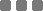## Thursday, November 28, 2013

### Math: Finding the Shortest Grid Paths Possibilities

This is a brain teaser, if you will, which somewhat agitated me at first. ^_^ Why? Because, well, I tried to solve "too many" grids for the beginning. It "wasted" a lot of my fun time. I got the answer, but tinkering the "formulation" of that was really, really frustrating, um, not really. Then, I searched and found cool resource which covered that Math teaser. Actually this one is pretty much old, lots of people have already posted how to solve this. I like it by the way. Anyway, I will give you the basic idea to solve this. This is like a maze, sort of. The starting and the finishing points are always separated diagonally in this thingy.

The rule is simple : we can't go other than using (following) the grid line, the border of each box.

First : finding the shortest path
Of course, the first thing to do is to find the shortest path to reach the destination. In this friggin' problem, we will always have to pick two ways (between four ways : up-down-right-left).

If we start from the top left, to go diagonally to the bottom right, we will have two options to move, those are : down or right. Other than those, we won't be going on the shortest path.

If we start from the bottom left, and we have to reach the top right, then we'll have two options, those are up or right.

For example, the 3x3 grid. The easiest way to find the shortest path is just counting the side border of it. I put different colors, green and red, so you can see them. In that example, we have 6 steps as our shortest path.

The last : using our brain
How many ways the shortest path arrangements can we build on that example? Now, right here, the brain teaser starts.

Basically, this is a combinatorics problem. So, like this, suppose those 6 steps (the shortest path we discovered) as the entire space (the set) consists of 6 elements. Then we only have two options to move to get that shortest path. In the first example above, we'll move 3 downs and 3 rights, or {D,D,D,R,R,R}. Or, in the second example, we'll move 3 ups and 3 rights, or {U,U,U,R,R,R}. Other than those moves, we can't have the shortest path. We just need to scramble those elements and find how many different combinations can be arranged. With using the formulation of combination, we'll have n=6, and k=3.
If we have different problem, for instance the size of shortest path is 13, and we can move 3 downs and 10 lefts, meaning 3 by 10 grid, then the n=13, and we can freely choose the k to be 10 OR 3, both will generate the same result. With simple Algebra we can find that the combination formula is symmetrical.
This is the general combination formulation : Actually, this one is multiset permutational stuff. We can also declare the formulation like this, the number of ways of arranging n objects, with multiple different number of types (subsets) - of p, q, r, etc :
 n! p!·q!·r!·...

Both methods will give us the exact same result.

Let's use the combination, we can just substitute those variables with our values :
6C3 = 6! / [ 3!·(6 - 3)! ]
= 6! / [ 3!·3! ]
= 120 / 6
= 20

And the answer is 20. We have 20 different arrangements possibilities for those two examples above.

The simple animation
Alright, this is a simple example of animating that problem (brain friggin teaser) with jQuery. I put 3 by 1 grid. So we have 4 steps for the shortest path, and we have 3 downs and 1 right, or {D,D,D,R}. Let's use multiset-permutation to solve this. So we have n = 4, and the subsets : p = 3 and q = 1.
4C1 = 4! / [ 3!·1! ]
= 4 / 1
= 4
We have four possibilities to move with the shortest path for this particular problem. Click and watch the demo below.

 A BMath: Finding the Shortest Grid Paths Possibilities
https://monkeyraptor.johanpaul.net/2013/11/finding-shortest-grid-paths.html